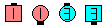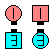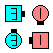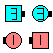Definitions of Square Dance Calls and Concepts
By Golly [C4]

\$B8~\$+\$\$9g\$C\$?%+%C%W%k\$+\$i(B. \$B\$3\$N%3!<%k\$O(B Sweep 1/4 \$B\$,

Flow \$B\$NJ}8~\$K6a\$\$?M\$,(B Sweep 1/4 \$B\$r\$7(B, \$BB>\$N?M\$O(B Dodge \$B\$r\$7(B Any Shoulder Wheel Thru \$B\$r\$7\$^\$9(B.

Mini Wave \$B\$N(B Box \$B\$G=*\$o\$j\$^\$9(B.

Recycle By Golly:Recycle By Golly\$B\$NA0(B Recycle\$B\$N8e(B Flow \$B\$NJ}8~\$K6a\$\$?M\$,(B Sweep 1/4,\$BB>\$N?M\$O(B Dodge\$B\$N8e(B \$B\$N8e(B Dodgers Any ShoulderWheel Thru\$B\$N8e(B (\$B=*\$o\$j(B)

Cheat #1: Flow \$B\$NJ}8~\$X(B Veer \$B\$r\$7\$F(B; Any Shoulder 1/2 Tag \$B\$r\$7\$^\$9(B.

Cheat #2: Sweep 1/4 \$B\$r\$7\$F(B; Sweep 1/4 \$B\$GDI\$\$\$+\$1\$F\$\$\$k?M\$,(B Diagonal Pull By (\$B30B&\$N \$BLu

C3B \$B\$G(B By Golly \$B\$NA0\$K;H\$(\$k%3!<%k\$N0lIt\$N%j%9%H(B (\$BLu

 Bend The Line ( 2-Faced Line \$B\$+\$i(B ) Ferris Wheel ( resulting Centers ) Sweep 1/4 Cross Cycle ( 2-Faced Line \$B\$+\$i(B ) Linear Cycle Turn & Deal ( \$B1& ) Cycle & Wheel Recycle ( Wave \$B\$+\$i(B ) Wheel & Deal ( 2-Faced Line \$B\$+\$i(B ) Fancy ( resulting Centers ) Shakedown

\$B%3!<%i!<\$N\$?\$a\$NCm

• \$B%3%^\$rF0\$+\$9\$H\$-(B, By Golly \$B\$O(B Touch 1/4 \$B\$^\$?\$O(B Left Touch 1/4 \$B\$H7k2L\$,F1\$8\$G\$9(B.
• Recycle By Golly \$B\$O(B Flip Back \$B\$H7k2L\$,F1\$8\$G\$9(B.
• Ceder Square Dance System (CSDS) \$B\$,;H\$&(B By Golly \$B\$NBeBX\$NDj5A(B: \$B8~\$+\$\$9g\$C\$?%+%C%W%k\$+\$i(B, \$BA4\$F\$N?M\$N(B Roll \$B\$NJ}8~\$,F1\$8\$H\$-(B (\$B\$9\$J\$o\$A(B, \$B\$b\$7l9g\$O(B Veer Left and 1/2 Tag \$B\$r(B, \$BB>\$N>l9g\$N(B Roll \$B\$NJ}8~\$,:8\$N>l9g\$O(B Veer Right and Left 1/2 Tag \$B\$r\$7\$^\$9(B. \$B\$3\$N0lHL2=\$5\$l\$?Dj5A\$K\$h\$j(B, By Golly \$B\$r(B Couple Up \$B\$d(B, Right & Left Thru \$B\$J\$I\$N%3!<%k\$N\$"\$H\$K;H\$&\$3\$H\$,\$G\$-\$^\$9(B.